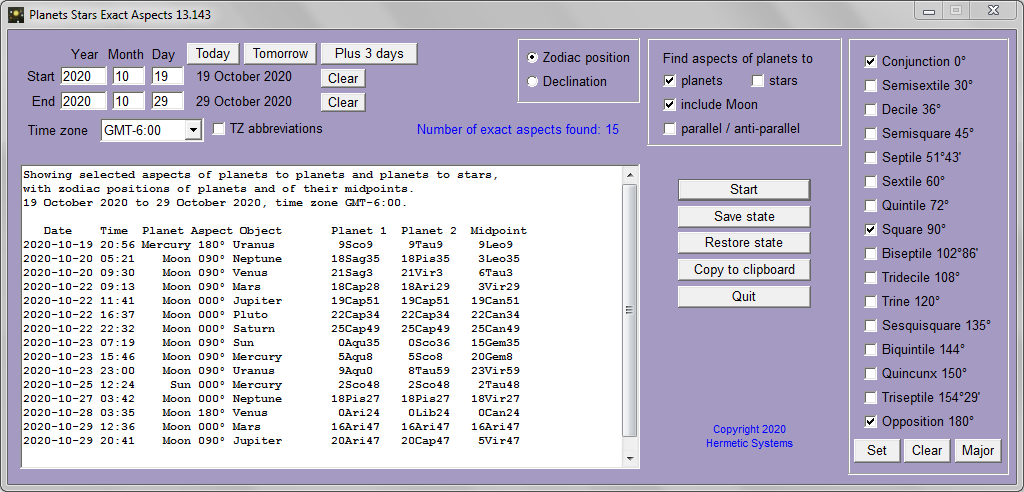# Planets Stars Exact Aspects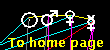## A Program to Calculate Exact times of Geocentric Aspects between Planets and Stars

An aspect between two celestial objects (here the Sun, the Moon, the planets and selected stars) occurs when there is a certain angle (named e.g. conjunction, opposition, trine, square, etc.) between them on the geocentric zodiac (or in other words, when the difference of their geocentric ecliptic longitudes is less than a specified amount, e.g. 5°). The aspect is then said to be in effect. A transit between two objects is said to be in effect when the angle between them remains within a certain range (e.g. 5°) over a period of time. This program does not calculate transits — rather it calculates the dates and times at which an aspect between two objects is exact.

Here is a typical example of its output: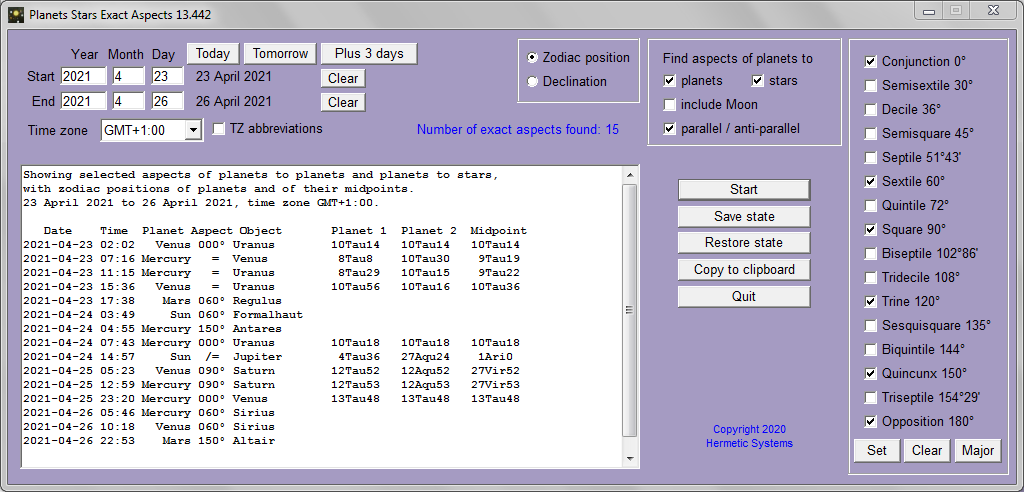The program can find the dates and times of 16 different aspects, as shown in the screenshot, not just the major aspects square, trine, etc.

It is possible to set the precision used when ascertaining whether an aspect involving a particular planet is exact by setting values in a side-panel, as shown in this screenshot. (That side-panel appears when the right border of the main panel is dragged to the right.) It is not recommended to change these values.

The first two zodiac positions are those of the two planets at the time of the exact aspect. The third is the zodiac position midway between those planets, called, appropriately, the midpoint.

When declination is selected, rather than zodiac position The output is: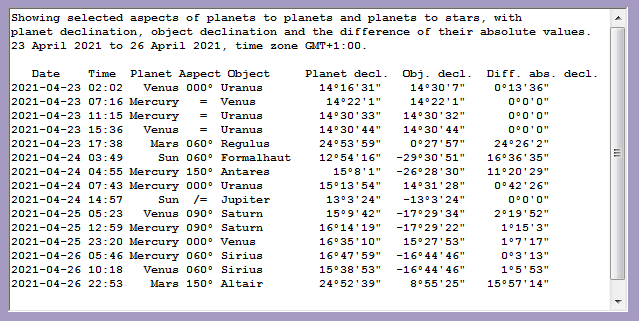The first two angle values in each line in the output box are the declinations of the planet and the second celestial object (planet or star). This term is defined by the Encyclopedia Britannica as follows:
Declination, in astronomy, is the angular distance of a body north or south of the celestial equator. Declination and right ascension, an east-west coordinate, together define the position of an object in the sky. North declination is considered positive and south, negative. Thus, +90° declination marks the north celestial pole, 0° the celestial equator, and -90° the south celestial pole.

The angle value in the third column, diff. abs. decl., is the difference between the absolute values of the angle values in the first two columns. When this is zero the two objects are said to be either parallel = or anti-parallel /= according to whether or not the sign (positive or negative) of the declinations of the two objects is the same or not.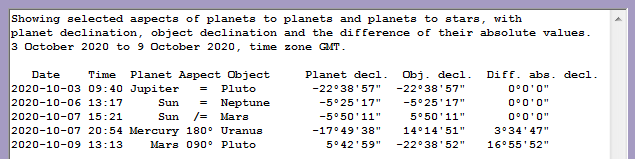The Moon moves very rapidly in comparison to the planets, so in one day it forms many exact aspects to them. Thus including the Moon will produce an output in which most of the lines show exact Moon aspects. If we are interested only in exactly when the Moon is conjunct, square to, or in opposition to the planets then we obtain an output such as: